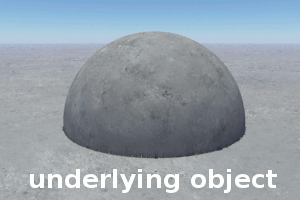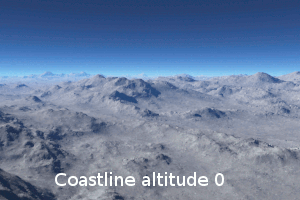# Difference between revisions of "Power Fractal Shader v3 - Displacement Tab"

Description:
Displacements are one of the two main function of a Power Fractal Shader. When displacement is enabled in this shader, the color information generated by the fractal are interpreted as elevations, where the high color equals maximum elevation, and the low color is the minimum elevation. Other shaders, such as the surface shader, can use this information to eventually apply structure to objects like the planet, fake stones, image maps, etc.

The elevation information is independent of the color chosen in the "Colors" tab of the Power Fractal Shader. In fact, colors can be completely disabled in the Power Fractal Shader and displacement information is still generated for use by other shaders and functions.

Settings:

• Apply displacement: If this is checked the shader will generate displacement. The popup list at right allows you to choose the direction that displacement is applied in. Any options in the popup list that have "(requires computed normal)" require that there be a Compute Terrain or Compute Normal connected somewhere above the node in the network to work properly. The popup has the following options:
• Along vertical: Displacement will happen along the normal of the underlying object (i.e. the planet or a model) without any displacement being applied.
• Along normal: Displacement will happen along the current surface normal.

• Vertical only (requires computed normal): Displacement only happens along the normal of the underlying object (i.e. the planet or a model). The displacement is scaled by the difference between the object normal and the surface normal. Displacement is reduced as the angle between the normals approaches 90°.

• Lateral only (requires computed normal): Displacement only occurs in the lateral plane, or in other words perpendicular to the normal of the underlying object.

• Lateral normalized (requires computed normal): This is the same as Lateral only but the normal is normalised (scaled so it has a length of 1).

• Displacement amplitude: If displacements are enabled in a power fractal, this value determines the amplitude of the displacement. For example, if the power fractal is used to generate a procedural terrain, the amplitude determines how high and low the maximum terrain features can get.

• Displacement offset: This value is added to the displacement values. This creates the effect of offsetting the displacement by a set amount along the Displacement direction. Positive values push the displacement out so it looks almost as if it was sitting on a plinth. Negative values will sink the displacement back into the surface. It doesn't reverse the displacement, it's more like creating a hole in the surface and then applying the displacement to the bottom of the hole.

• Displacement roughness: This slider allows you to adjust the roughness of the displacements. Values less than 1 reduce the roughness of the displacement.

• Displacement spike limit: This setting helps with reducing spikes which occur in the displacement. Especially when the fractal is used for generating realistic terrains, reducing both the spikes and roughness come in handy.

• Continue spike limit: TBC

• Adjust coastline: When checked, "adjust coastline" will smooth the displacements by the two following parameters

• Coastline altitude: Set the altitude below which the smoothing begins.

• Coastline smoothing: Adjust the smoothing factor.

Example Movies

 Displacement directionDisplacement amplitudeDisplacement offsetCoastline altitudeCoastline smoothingBack to: Power Fractal Shader v3

Literally, to change the position of something. In graphics terminology to displace a surface is to modify its geometric (3D) structure using reference data of some kind. For example, a grayscale image might be taken as input, with black areas indicating no displacement of the surface, and white indicating maximum displacement. In Terragen 2 displacement is used to create all terrain by taking heightfield or procedural data as input and using it to displace the normally flat sphere of the planet.

A shader is a program or set of instructions used in 3D computer graphics to determine the final surface properties of an object or image. This can include arbitrarily complex descriptions of light absorption and diffusion, texture mapping, reflection and refraction, shadowing, surface displacement and post-processing effects. In Terragen 2 shaders are used to construct and modify almost every element of a scene.